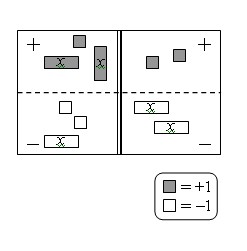Home > AC > Chapter 3 > Lesson 3.1.3 > Problem3-29

3-29.

Write the equation represented in the equation mat below. 3-29 HW eTool (CPM). Homework Help ✎1. Simplify as much as possible and solve for $x$.

2. Evaluate both the left side and the right side using your solution from part (a). Remember that if your solution is correct, both sides should have the same value.

For more help solving this problem refer to problem 2-104.

Use the eTool below to help solve the problem.
Click the link at right for the full version of the eTool: AC 3-29 HW eTool